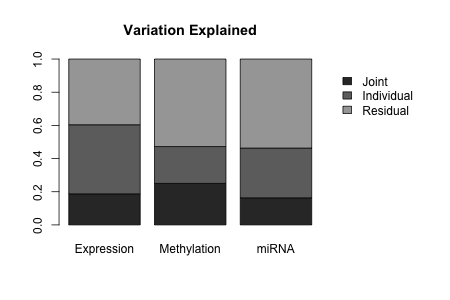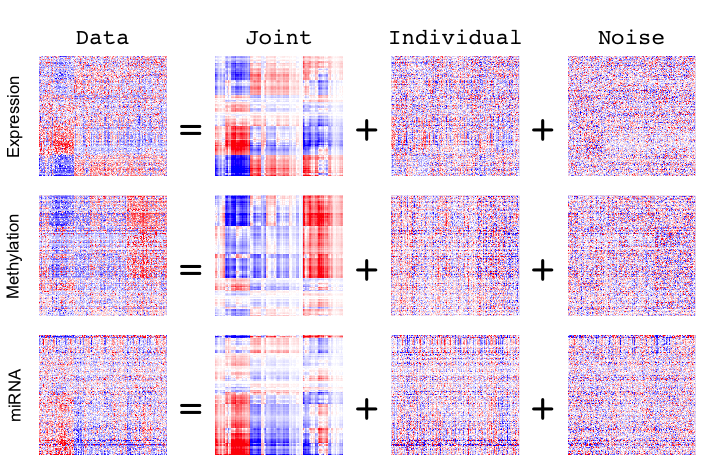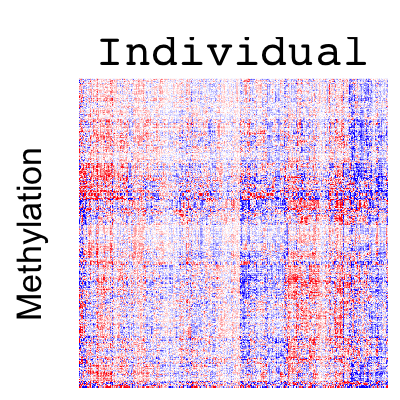# Exploration of multi-source BRCA data using r.jive

#### 2020-11-11

This vignette illustrates the usage and capabilities of the r.jive package, using publicly available data on breast cancer (BRCA) tumor samples from The Cancer Genome Atlas. This package implements Joint and Individual Variation Explained (JIVE), a flexible exploratory method for the integrated dimension reduction and visualization of multiple high-throughput data sources measured on the same samples set. The method was originally described in E. Lock et al. (2013), and the package is described in O’Connell and Lock (2016); users should refer therein for details. Briefly stated, JIVE decomposes a multi-source dataset into three terms: a low-rank approximation capturing joint variation across sources, low-rank approximations for structured variation individual to each source, and residual noise. This decomposition can be considered a generalization of Principal Components Analysis (PCA) for multi-source data.

Here we avoid methodological details and simply describe the functionality of the R package with a data example.

## Loading the data

First, if you have not done so already, install and load the package via CRAN:

``````install.packages("r.jive")
library(r.jive)``````

The package requires two dependencies: SpatioTemporal for inputing missing values, and gplots for some plotting functions.

To load the BRCA data, enter
`data(BRCA_data)`
These data consist of a single list object `Data` that contains the data. The list has one entry for each of three different molecular sources:

• `Data[]` (`Data\$Expression`): gene expression matrix for 654 genes (rows) and 348 samples (columns)
• `Data[]` (`Data\$Methylation`): DNA methylation matrix for 574 cg sites (rows) and 348 samples (columns)
• `Data[]` (`Data\$miRNA`): miRNA expression matrix for 423 cg sites (rows) and 348 samples (columns).

The 348 columns are shared by the data sources (here, they correspond to tumor samples), and must be in the same order. This is the general data format recognived by `jive`.

These data were originally obtained from the data freeze for TCGA’s flagship BRCA publication (Cancer Genome Atlas Network (2012)). The data were filtered and processed as described in E. F. Lock and Dunson (2013). E. F. Lock and Dunson (2013) describe an analysis of these data to identify jointly present sample clusters, and these clusters are loaded here as the vector `clusts`. Here we will only use the cluster labels to visualize and interpret results.

## JIVE estimation

The default function to estimate the JIVE decomposition is `jive`. This function involves several options, including the capability to input given ranks for joint and individual structure or to use a method to estimate these ranks. For more information on available options, enter `?jive`.

Two algorithms to select the ranks are included as options. One is a permutation-based approach described in E. Lock et al. (2013), the other is a BIC-motivated approach. We find that the accuracy of permutation estimated ranks are generally better, but BIC is less computationally intensive. By default the ranks are selected via permutation, with row-orthogonality enforced between the joint and invidual estimates and also between each individual estimate. Previously orthogonality was only enforced between joint and individual estimates, but we find that also enforcing the individual estimates to be orthogonal to each other improves convergence and robustness of the results to rank mispecification.

We estimate the decomposition on the BRCA data with these and other defaults. Note that the results below differ slightly from the results described in O’Connell and Lock (2016), as the default algorithm has been improved.
(This can take some time to complete, about 15 min, to compute the same estimates more quickly with given ranks see below.)

`Results = jive(Data)`

Estimating joint and individual ranks via permutation…
Running JIVE algorithm for ranks:
joint rank: 2 , individual ranks: 21 14 20
JIVE algorithm converged after 113 iterations.
Re-estimating joint and individual ranks via permutation…
Running JIVE algorithm for ranks:
joint rank: 2 , individual ranks: 27 25 25
JIVE algorithm converged after 95 iterations. Re-estimating joint and individual ranks via permutation…
Running JIVE algorithm for ranks:
joint rank: 2 , individual ranks: 27 26 25
JIVE algorithm converged after 98 iterations. Re-estimating joint and individual ranks via permutation…
Final joint rank: 2 , final individual ranks: 27 26 25

The output gives the ranks used for each call to the base JIVE algorithm (here, 4 calls) and the number of iterations until convergence for each call. The method ends when the ranks are the same for two consecutive calls. For this example, the chosen ranks are

• rank 2 joint structure
• rank 27 structure individual to gene expression
• rank 26 structure individual to methylation, and
• rank 25 structure individual to miRNA.

The results are given as an object of the S3 class JIVE, with values including

• `Results\$data`: A list of the three data matrices used for JIVE estimation. These are centered and scaled version of the original data matrices (by default, each data matrix is scaled by its total variation).
• `Results\$joint`: A list of three data matrices giving estimated joint structure for each source.
• `Results\$indiv`: A list of three data matrices giving estimated individual structure for each source.

Each list is labeled with the names given in the original input data (e.g., `Results\$data\$Expression` gives the scaled expression data). These are also used as labels for figures that depict the JIVE results.

To simply estimate the JIVE decomposition with the ranks determined above, enter
`Results = jive(Data,method="given",rankJ=2,rankA=c(27,26,25))`
these results are identical to those obtained after running the method with rank selection.

## Visualization and interpretation

The r.jive package provides three functions that generate figures with very different views of the JIVE results, and we briefly illustrate each function here. All three take an object of class JIVE as input, with additional optional parameters.

The most simple function is `showVarExplained`, which displays a barchart of the amount of variation explained by joint and individual estimates in each data source. Here we save the resulting figure for the BRCA data as a .png file, shown below.

``````png("VarExplained.png",height=300,width=450)
showVarExplained(Results)
dev.off()``````The variation explained by joint structure is higher than that for individual structure for methylation data, despite the much higher rank of individual structure (rank 26 individual vs. rank 2 joint for methylation). The estimated joint structure explains slightly less variability, but still a substantial proportion of variability, in the gene expression and miRNA data.

We can display the actual JIVE estimates, in the form of low-rank matrix approximations, as heatmaps using the `showHeatmaps` function. By default, this will create heatmaps of the full JIVE decomposition (Data=Joint+Individual+Residual) and order the rows and columns of all matrices by complete linkage clustering of the joint structure. For larger datasets, performing the clustering and rendering the images can take some time; for these data the process takes less than a minute. You may need to fiddle with the image dimensions a bit for the results to look nice (if the dimensions are too small, the text may be cut-off).

``````png("HeatmapsBRCA.png",height=465,width=705)
showHeatmaps(Results)
dev.off() ``````The columns (samples) are vertically aligned for all heatmaps, with red correspoding to higher values and blue lower values. The estimated joint structure shows clear joint patterns that can also be seen in three of the original data heatmaps. However, note that the joint structure appears less prominently in the miRNA data heatmap, as the joint structure explains less of the miRNA variability.

In addition, the `showHeatmaps` function includes options to specify how to order rows and columns, and which matrices to display; for details enter `?showHeatmaps`. For example, we can order by the individual methylation structure (data source 2) and show only this heatmap.

``````png("HeatmapIndivMeth.png",height=400,width=400)
showHeatmaps(Results,order_by=2,show_all=FALSE)
dev.off() ``````alt text

One factor appears to be a mean effect, distinguishing those samples with relatively high methylation genome-wide from those with relatively low methylation.

To further examine the biological relevance of the estimated joint structure, we consider the “point cloud” view provided by the `showPCA` function. This shows the patterns in the column space that maximize variability of joint or individual structure, analogous to principal components. Any number of components from the joint or different individual structure estimates can be shown in multi-panel scatterplots. First, we view the two components of joint structure (note: because the joint structure is rank 2, it has only 2 principal components). We color these components by the clusters defined in the `clusts` variable.

``````Colors = rep('black',348)
Colors[clusts==2] = 'green'
Colors[clusts==3] = 'purple'
png("JointPCA.png",height=400,width=400)
showPCA(Results,n_joint=2,Colors=Colors)
dev.off()``````